Publication date: 08/13/2020

## Control Limits for XBar and R Charts

JMP generates control limits for XBar and R charts as:

LCL for XBar chart =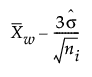UCL for XBar chart =LCL for R chart =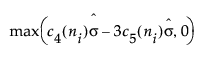UCL for R chart =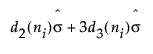Center line for R chart: By default, the center line for the ith subgroup (where 3 is the sigma multiplier) indicates an estimate of the expected value of Ri. This value is computed as: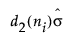, where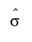is an estimate of σ.

The standard deviation for XBar and R charts is estimated by: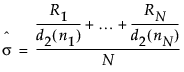where: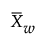= weighted average of subgroup means

σ = process standard deviation

ni = sample size of ith subgroup

d2(n) is the expected value of the range of n independent normally distributed variables with unit standard deviation

d3(n) is the standard deviation of the range of n independent normally distributed variables with unit standard deviation

Ri is the range of ith subgroup

N is the number of subgroups for which ni ≥ 2.